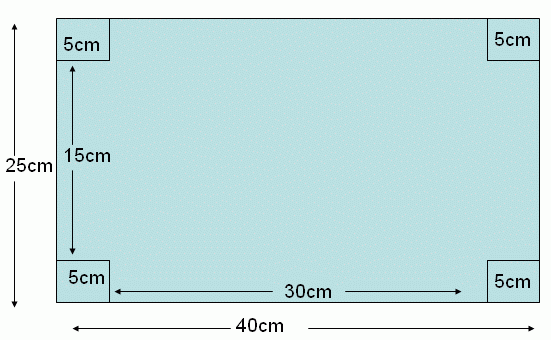Email us to get an instant 20% discount on highly effective K-12 Math & English kwizNET Programs!

#### Online Quiz (WorksheetABCD)

Questions Per Quiz = 2 4 6 8 10

### Grade 7 - Mathematics Word Problems on Cuboid - I

 Example: The length and width of a rectangular metal sheet are 40 cm and 25 cm respectively. Four squares each of side 5 cm are cut and removed from four corners of the sheet, and the remainder is folded to form a cuboid(without the top face). Find the volume. Solution:In the given rectangular sheet has length and breadth equal to 40 cm and 25 cm respectively. If four corner squares of the side 5 cm are removed and rest is folded into a cuboid. Then the length and width of the cuboid is reduced by twice the side of square. Therefore the length of the cuboid = 40 - (2 * 5) = 40 - 10 length (l)= 30 cm. The width of the cuboid = 25 - (2 * 5) = 25 - 10 width (b) = 15 cm. The height of the cuboid itself is equal to the side of the square removed. Therefore the height of the cuboid = 5 cm. Hence the volume of the cuboid is V = l * b * h V = 30 * 15 * 5 Volume = 2250 cubic centimeters. Directions: Read the above example carefully and answer the following questions. Also make 10 different examples of your own and illustrate each by making cuboids.